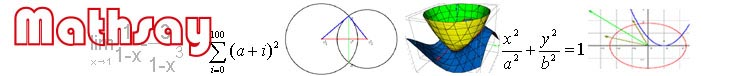Home

Support

Examples

Geometry

Trigonometry

Coordinate geometry

Function

Equation

Vector

3D geometry

3D plot

Limit & derivative

Math symbol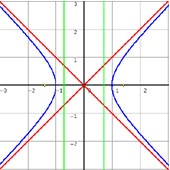Know hyperbola. Show its directrixs,  asymptotes and e. F1 and F2 are focus.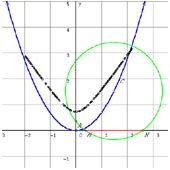Circle C pass through point A(0,p), p>0. The center of the circle is C. C moves in curve x2=2py. MN is the chord of C cut by X axis. Dynamically show the length of MN.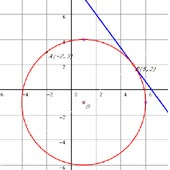Know a circle passes point A(12,3),and its tangent line is straight line 4x+3y-26=0, tangent point is (5,2).Find the circle equation.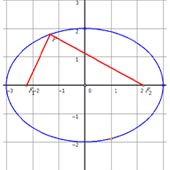Ellipse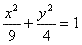, its focuses are F1 and F2, P is point which can be moved on ellipse. When